# Training

 Problem 1 If neuron weights are seen as a vector of length (vector norm) one as shown in the figure, proof that the output of each neuron is equal to the cosine of the angle measured between the input vector and the neuron weights vector. See figure below. Observe that the same result must be extended to any vector size, that is the same holds true if the vectors are in a N-dimensional space.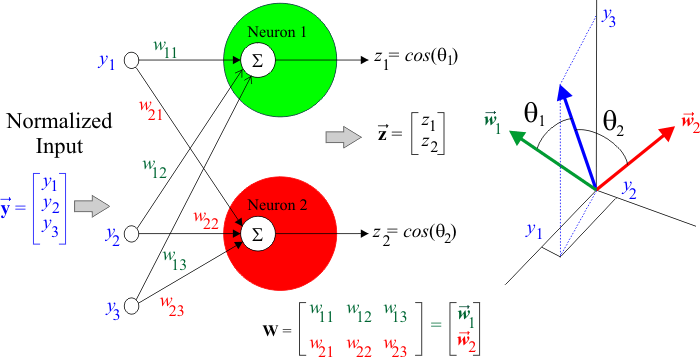Problem 2 Suppose that the input of a Kohonen network is a vector v of size N. Suppose also that the network has two neurons: A and B. Neuron A has a weights vector almost in the same direction as the vector v. Neuron B has a weights vector that makes an angle of 40 degrees with the vector v. Which neuron will produce a higher output?

## Competitive Learning

A network that operates with competitive learning allows only some neurons to learn. Thus, neurons compete with each other for the privilege to learn. In some cases, only one neuron (called Winner) is allowed to learn at each time. In other cases, a group of neurons is allowed to learn. In this later case, one neuron may be allowed to learn a lot while close neurons may be allowed to learn little.

## Kohonen Network Training

A Kohonen network is trained using competitive learning. During training, each case in the training set is presented to the network, the neuron with the highest activation (the winner) will adjust its weights; the weights of the remaining neurons will remain the same. At each iteration, the weights of the winner are updated so that they will react to some particular training cases. Each neuron will react to a subset of training cases, meaning that each neuron will specialize to a set of the training cases that are close to each other. If training is successful, neurons weights will eventually converge, and no further training will change the weights. At this point, training is completed. This is shown in the figure below where input vectors are shown gray, while neuron weights are displayed in green and red. First, the weights vectors are randomly placed. As training progresses, the angle between a neuron vector and some input vectors are being reduced to create clusters. At the end of the training, each neuron vector will be located in the center of a cluster or input vectors.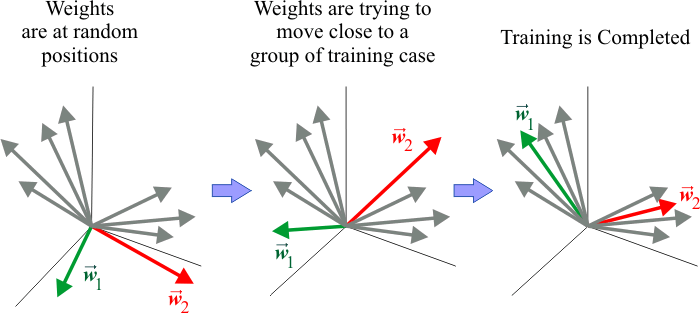Tip The most important aspect of Kohonen networks is that their inputs and weights must be normalized to have a length of one.

## Learning Rate

One of the most important parameter of unsupervised training is the learning rate. It is typically represented by the Greek letter alpha. The learning rate must be less than one. A value close to one, fast learning, is not recommended as there is the risk that the weights will never converge. A value close to zero will make convergence slow. A typical value is 0.4 or less. In some cases, it is recommended to reduce the learning rate, once the convergence of the weights is stable.

## Weights Update

From one iteration to the next, only the weights of the neuron with the highest activation (the Winner) will be updated. There are two common methods to update the weights of a Kohonen network: Additive Training or Subtractive Training. Both methods should be tried to solve a specific problem.

This method has two steps. First, a fraction of the input vector is added to the weight vector of the winner. Second, the neuron weights of the winner are normalized to have a length (vector norm) of one. The figure below shows how additive training works, each time a fraction of the input vector is added to the neuron weights, the neurons weights are pulled into the direction of the input vector.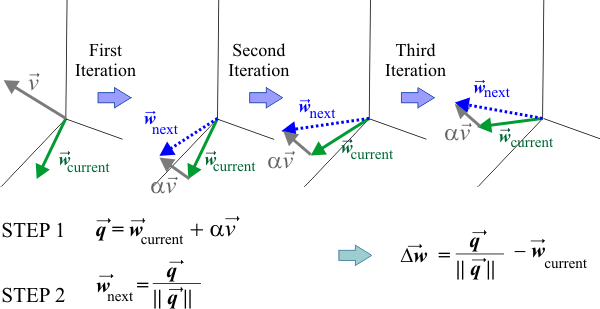## Subtractive Training

This method has also two steps. First, the subtraction vector, between the input vector and the winner vector is computed. Second, a fraction of the subtraction vector is added to the weights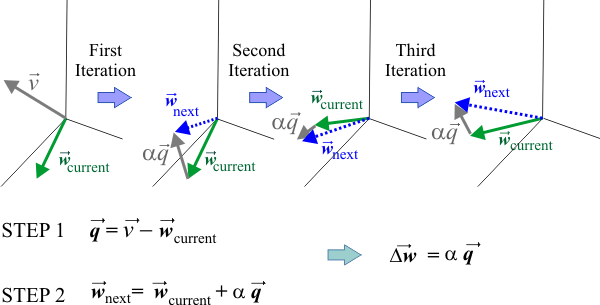Tip You may update the weights after presenting each training case to the network input, or may be update the weights after presenting all training cases to the network input. Some people are in favor of the first method. Other argue that the second method is more stable.

 Tip When using Additive training, it is not recommended to update the weights after presenting each training case, but to update the weights after presenting to the network input all training cases. Thus, weights are updated at the end of each iteration increasing the probability of a more stable convergence.

 Problem 3 Discuss the advantages and disadvantages of using the maximum error versus the mean squared error when reporting optimization results.

## Maximum Error

In competitive learning, the mean squared error is not used as it does not clearly represent the performance of the network. Instead, Kohonen networks use the maximum error to analyze the performance of a network.

## Loser Neurons

In competitive learning, each neuron competes for learning, making very likely that some neurons will never have a chance to learn. These neurons are called loser neurons. As loser neurons do not perform any actual work, they are not desirable. Thus, in competitive learning there must be a mechanism to prevent a neuron to win too many times, and help loser neurons to learn. There are several strategies, for instance, it is possible to penalize a neurons each time it wins. There are, or course, several strategies with their advantages and disadvantages.

 Problem 4 A Kohonen network has three inputs and two ouputs as shown. The training set has only two cases as shown in the figure. Compute the output and the winner neuron. (a) For the first training case. (b) For the second training case.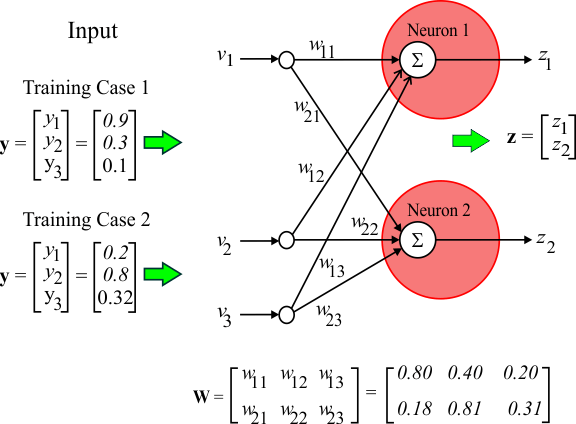Problem 5 In the previous problem, compute the network weights for the following iteration using a learning rate (alpha) of 0.25. (a) Using Additive Training. (b) Subtractive Training.Problem 6 Indicate whether the following statement is true or false: In a Kohonen network, there is no rejection class, that is, the network must assign a class to all input cases. This is the essence of competitive learning, there is always a winner.

 Problem 7 Indicate whether the following statement is true or false: In a Kohonen network, input cases are grouped by their classes. If an input case produces a very small output in the winner neuron, this implies that this input case is not properly represented by this neuron. That is, this input case may deserve a neuron by itself.

 Problem 8 Indicate whether the following statement is true or false: In a Kohonen network, if an input case is not properly represented by any neuron; then this input case must be used as the weights of one neuron to force this neuron to represent this case.

 Problem 9 Indicate whether the following statement is true or false: In a Kohonen network, if an input case produces a very similar output in two neurons, then it can be said that one of these two neurons is useless. Thus, it is recommended to force one of these neurons to represent other training cases.

 Problem 10 Indicate whether the following statement is true or false: A Kohonen network learns without supervision. This implies that the user may or may not have a way to tell the network what features are important. As a matter of fact, the network will use its own rules to classify any input, and the ANN classifications may differ from what the user think are the correct ones.

 Problem 11 Indicate whether the following statement is true or false: In a Kohonen network it is possible to use a confusion matrix to evaluate the performance of the network.